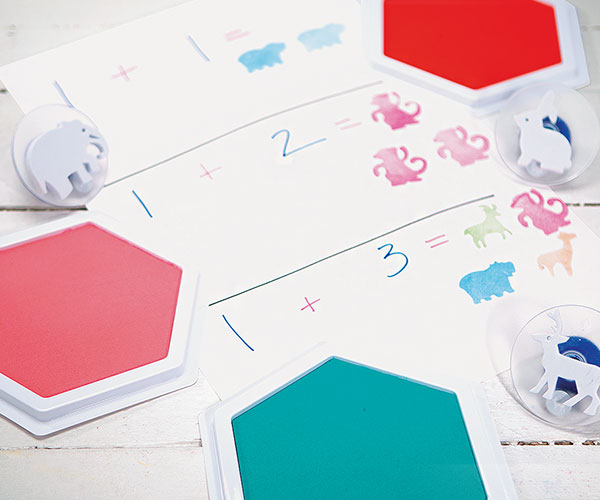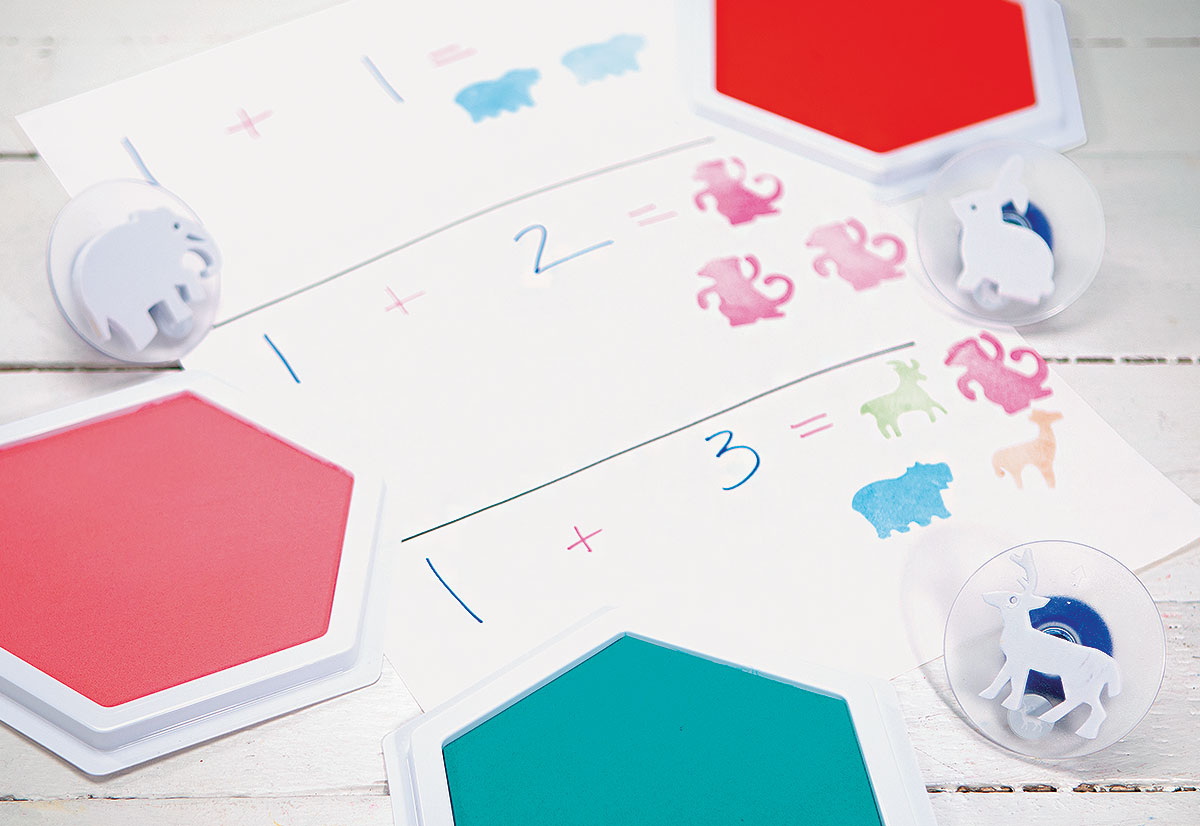< Browse Activities

# Stamping Math# Stamping Math## Intro

Use stamping to reinforce simple math skills! This engaging activity combines the artistic aspects of stamping with addition and/or subtraction to build one-to-one correspondence, number recognition and other critical early math skills.

## Set Up Ideas

For beginners, you may have children use the same object for each numeral. For more advanced math, have the children use multiple objects. For example, 1 + 2 could be shown by 1 monkey + 2 hippos.

## Let’s Get Started

Gather the following materials: small object or animal stampers, construction paper and washable stamp pads. You may also need markers or crayons. This activity is easy to vary in several ways. Children may use both numeral and object stampers, or just one or the other.

## STEP 1

Create simple math worksheets with small number equations such as 1+1, 1+2, etc. Use numbers appropriate for the children's skill level.

## STEP 2

Leave space at the end of each equation and underneath the equation for kids to stamp animals below each numeral.

## STEP 3

Kids will use an object to stamp each of the numbers in the equation, and then count the total that they just stamped and stamp the final answer after the equal sign.

## STEP 4

Children can check their work by counting the number of animals at the end of the equation and those before the equal sign.

This activity can help children grasp one-to-one correspondence through stamping. Children may wish to use counters to find the correct number of objects represented by the numeral before stamping objects or animals onto the sheet.## Tips and Tricks

To save time, you might consider creating a template for your math worksheet that you can easily copy. Simply keep in mind whether the children will use numeral stampers, object stampers or both.## Follow up Activity

After you've done addition with stampers, try subtraction! Follow the same process as above, only this time have the children stamp the correct number of fewer animals after the equal sign.

Comments are held for moderation, thank you.